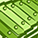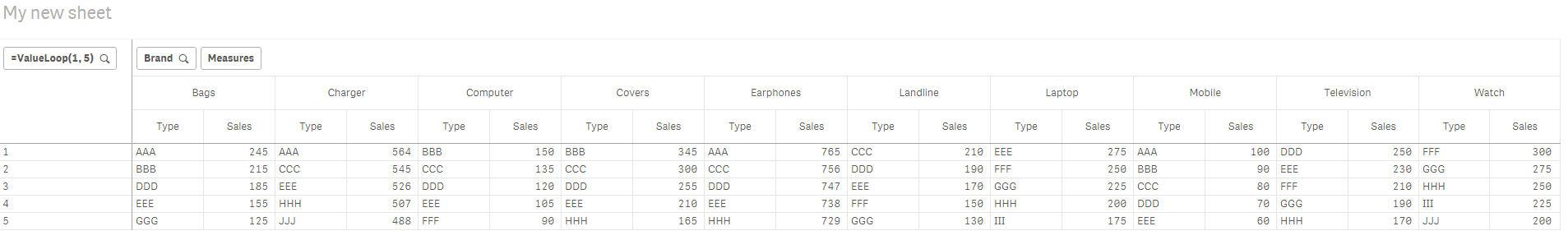New to Qlik Sense

If you’re new to Qlik Sense, start with this Discussion Board and get up-to-speed quickly.

Announcements
QLIKWORLD LIVE! MAY 16 - 19TH, EARLY BIRD DISCOUNTS! REGISTER TODAY
cancel
Showing results for
Did you mean:Contributor III

Top Ranks based on sales w.r.t Brands and Type in Pivot Table in Qlik Sense.

Hello Qlik Experts,

I have the following data in a table format and want to find out the Top Ranks based on Sales w.r.t Brands and Type in Pivot Table.

I require the output in a Pivot Table.

I am attaching a sample data Below in Excel format, where in I have to find the Top 5 Ranks of each Brands based on their Types and Sales.

The Input Data and the Output format is displayed in the Excel Workbook in different sheets.

1 Solution

Accepted SolutionsMVP

Bottom chart can be the other way you can do this... but then it looks different from what you needDimensions

Type

Brand

Expression

Aggr(If(Rank(Sum(Sales)) < 6, Sum(Sales)), Brand, Type)

7 RepliesPartner

HI,

Satish find the attached QliksenseApp

may it full fill your requirement. I used below expression for the dimension.

=Aggr(If(Rank(SUM(Sales)) <= 5, Type), Brand,Type)MVP

This?Dimensions

=ValueLoop(1, 5)

Brand

Expressions

Pick(ValueLoop(1, 5),

Aggr(NODISTINCT If(Rank(Sum(Sales)) = 1, Type), Brand, Type),

Aggr(NODISTINCT If(Rank(Sum(Sales)) = 2, Type), Brand, Type),

Aggr(NODISTINCT If(Rank(Sum(Sales)) = 3, Type), Brand, Type),

Aggr(NODISTINCT If(Rank(Sum(Sales)) = 4, Type), Brand, Type),

Aggr(NODISTINCT If(Rank(Sum(Sales)) = 5, Type), Brand, Type))

Pick(ValueLoop(1, 5),

Aggr(NODISTINCT If(Rank(Sum(Sales)) = 1, Sum(Sales)), Brand, Type),

Aggr(NODISTINCT If(Rank(Sum(Sales)) = 2, Sum(Sales)), Brand, Type),

Aggr(NODISTINCT If(Rank(Sum(Sales)) = 3, Sum(Sales)), Brand, Type),

Aggr(NODISTINCT If(Rank(Sum(Sales)) = 4, Sum(Sales)), Brand, Type),

Aggr(NODISTINCT If(Rank(Sum(Sales)) = 5, Sum(Sales)), Brand, Type))Contributor III
Author

Thanks Sunny,

However if I have to find the Values for Top 10, 20, 50 and so on, will I have to Have the Same equation in a longer format incrementing the Numbers.MVP

Well, I am not sure if there is another way... did you check out what arunaerra‌ posted... I am not sure what they have... but may be that works better for you...Contributor III
Author

Yes I checked what Aruna posted, however That doesn't solve my problem.MVP

Bottom chart can be the other way you can do this... but then it looks different from what you needDimensions

Type

Brand

Expression

Aggr(If(Rank(Sum(Sales)) < 6, Sum(Sales)), Brand, Type)Contributor III
Author

Thanks a lot SunnyTags
Community Browser